Wacker Art Basic FunctionsBild: "Kellerwald"

Mathematical Functions - Basic Functions

Taylor Series of the Exponential Function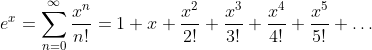Even Part of the Exponential Function - The Hyperbolic CosineA function is called even if f(x) = f(-x)

Odd Part of the Exponential Function - The Hyperbolic SineA function is called odd if f(x) = -f(-x)

Taylor Series of the Hyperbolic Cosine FunctionTaylor Series of the Hyperbolic Sine FunctionRelation between Hyperbolic Cosine Function and Cosine Function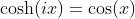Relation between Hyperbolic Sine Function and Sine FunctionTaylor Series of the Cosine FunctionTaylor Series of the Sine Function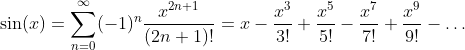Basic RelationsDefinition of Sine and Cosine Functions with the Complex Exponential FunctionDefinition of Tangent and Cotangent Functions with Sine and Cosine Functions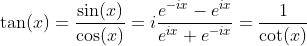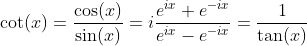Hyperbolic Tangent and Hyperbolic Cotangent FunctionsLogarithm Function: y = ln(x)

The logarithm function is defined for x values in the range 0 < x < ∞.

Taylor Series

Radius of convergence: |x| < 1 and x = 1.Definition with arctanhIntegral of ln(x)Derivative of ln(x)Further Properties of ln(x)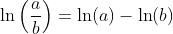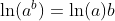Logarithm Functions loga(x) with Different BasesLogarithm loga(x) with Different Bases a

 a = 1/2 a = 2 a = e a = 10

Integral of ln(x)Function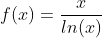This function can be used for the approximation of the prime counting function π(x).This relation is also known as prime number theorem.

Special FunctionDerivativeTaylor SeriesLogistic Function: y = 1/(1 + e-x)

Formulars of the logistic function: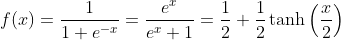The logistic function is the solution of the logistic differential equation.

Integration of the Logistic Function

Using the substitution u = 1 + ex and u' = ex gives du = exdx.Derivative of the Logistic Function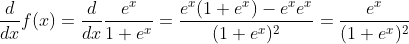Bernoulli FunctionThe Bi are the Bernoulli Numbers.

Bernoulli Numbers

The Bernoulli numbers Bi and Bernoulli numbers Bi* differ only in the value of i= 1. B1=-1/2 and B1* = 1/2, where they only have different sign. All the other values are the same.

 i 0 1 2 3 4 5 6 7 8 9 10 11 12 ... Bi 1 ±1/2 1/6 0 -1/30 0 1/42 0 -1/30 0 5/66 0 -291/2730 ...

Taylor SeriesRelationsInverse Hyperbolic Sine Function - Area Sinus Hyperbolicus: y = arsinh(x)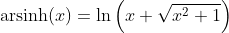Taylor SeriesRelationsInvers Hyperbolic Cosine Function - Area Cosinus Hyperbolicus: y = arcosh(x)Hyperbolic Tangent Function - Tangens Hyperbolicus: y = tanh(x)Inverse Hyperbolic Tangent Function - Area Tangens Hyperbolicus: y = artanh(x)Hyperbolic Cotangent Function - Cotangens Hyperbolicus: y = coth(x)Inverse Hyperbolic Cotangent Function - Area Cotangens Hyperbolicus: y = arcoth(x)Sine Function: y = sin(x)Inverse Sine Function - Arcus Sine: y = arcsin(x)Cosine Function: y = cos(x)Inverse Cosine Function - Arcus Cosine: y = arccos(x)Tangent Function: y = tan(x)Inverse Tangent Function: y = arctan(x)Cotangent Function: y = cot(x)Invers Cotangent Function: y = arccot(x)Cube Root Function: y = x1/3

Next Page:

06. März 2021 Version 2.0
Copyright: Hermann Wacker Uhlandstraße 10 D-85386 Eching bei Freising Germany Haftungsausschluß